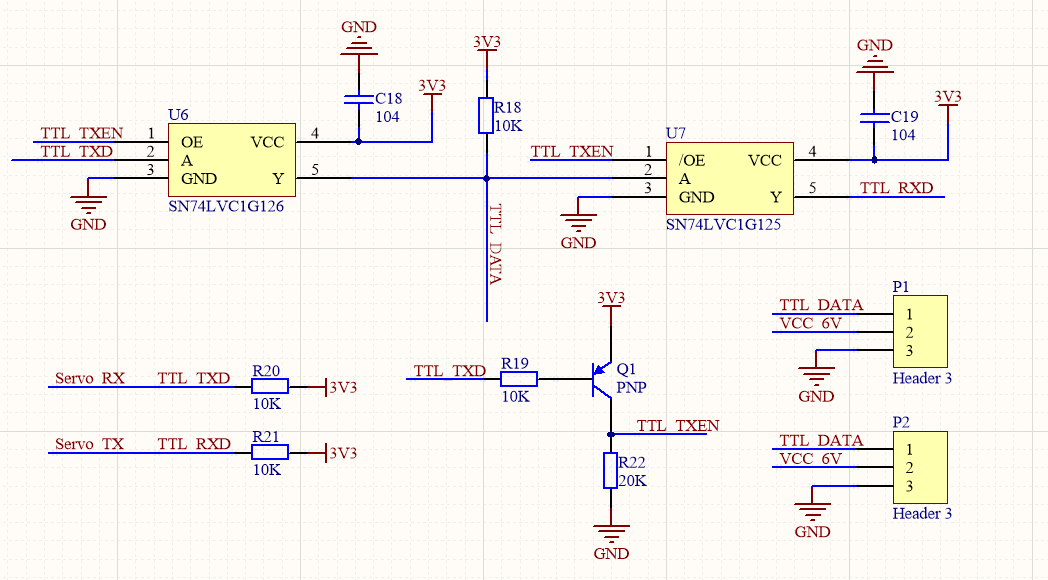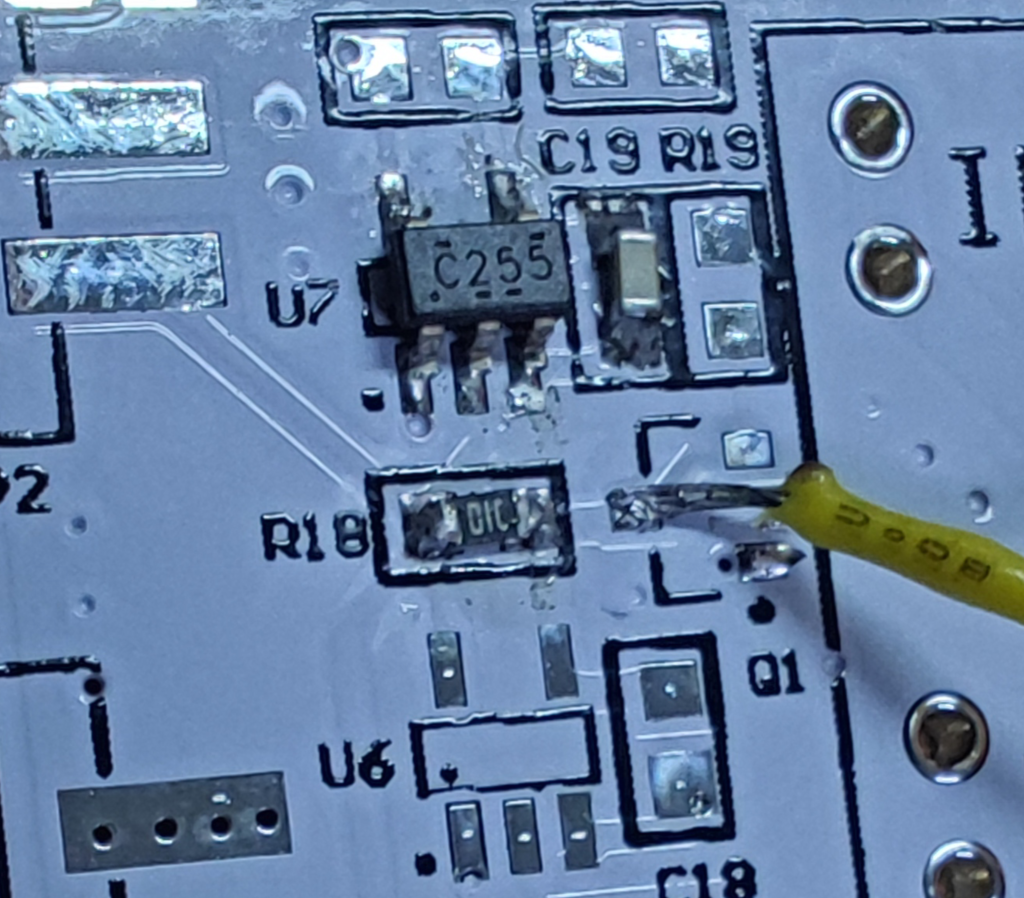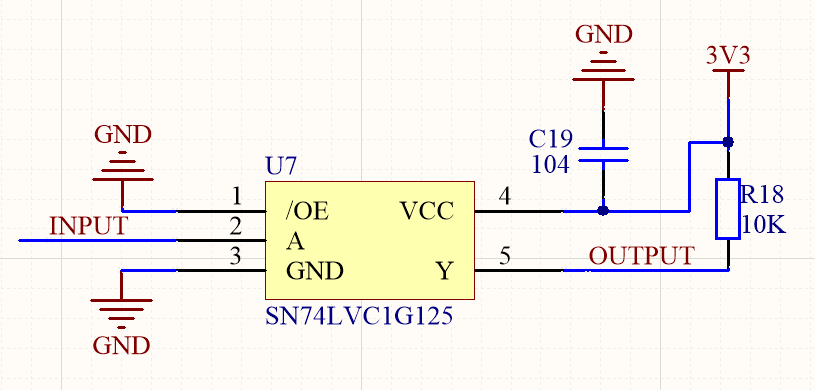# SN74LVC1G125: Output voltage can't go low

Part Number: SN74LVC1G125

Hi Team：

I didn't notice a minimum application circuit for SN74LVC1G125 in the datasheet, does it have one? Cuz when I test it separately( with a 0.1uF bypass capacitor near the power pin ), the output didn't go as I thought.

I powered SN74LVC1G125 with 3.3V, and connected /OE pin to GND. The results are as followed:

When the input is 3.3V, the output is 3.3V, this is correct.

but when the input is 0V, the output is about 2.4V. doesn't it should be 0V?

I've tested several SN74LVC1G125s and the results are all the same. They work perfectly fine in a pre-made PCB, so I must missed something.

### 5 Replies

• Hello, and welcome to the forums!

Yes, the output will be 3.3V when the input is 3.3V (assuming the supply is 3.3V), and the output will be 0V when the input is 0V (assuming GND is 0V).

Can you provide a schematic for your test circuit and/or an image of the circuit?

#### The Logic Minute training page has videos on many interesting topics that all are kept shorter than 5 minutes.

• In reply to Emrys Maier:

Hi and thanks for your time,

I drew a PCB with a SN74LVC1G12x circuit shown in the schematic below:but it won't work. So I simplified the circuit and used a part of the PCB to pinpoint the problem :and I found that the output is about 2.4V when the input is connected to GND. Can the circuit above work? If so, why the output wasn't 0V?

Thanks